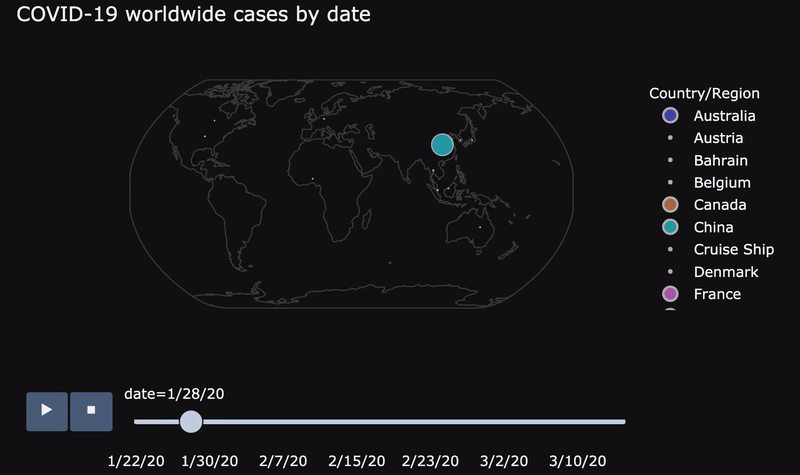# Analyzing COVID‐19 Outbreak

Last updated: May 28th, 2020# Analyzing the epidemiological outbreak of COVID‐19¶

A visual exploratory data analysis approach.

In [ ]:
import numpy as np
import pandas as pd
import matplotlib.pyplot as plt
import matplotlib.ticker as ticker
import seaborn as sns
import plotly.express as px
import theme

%matplotlib inlineWe will load COVID-19 data from the GitHub data repository for the 2019 Novel Coronavirus Visual Dashboard operated by the Johns Hopkins University Center for Systems Science and Engineering (JHU CSSE). Also, Supported by ESRI Living Atlas Team and the Johns Hopkins University Applied Physics Lab (JHU APL).

This data is daily-updated, so we can keep our project up-to-date just by loading this data again.

Let's load the data and quickly analyze it's columns and values:

In [ ]:
COVID_CONFIRMED_URL = 'https://raw.githubusercontent.com/CSSEGISandData/COVID-19/master/csse_covid_19_data/csse_covid_19_time_series/time_series_covid19_confirmed_global.csv'

print(covid_confirmed.shape)


In [ ]:
COVID_DEATHS_URL = 'https://raw.githubusercontent.com/CSSEGISandData/COVID-19/master/csse_covid_19_data/csse_covid_19_time_series/time_series_covid19_deaths_global.csv'

print(covid_confirmed.shape)


In [ ]:
COVID_RECOVERED_URL = 'https://raw.githubusercontent.com/CSSEGISandData/COVID-19/master/csse_covid_19_data/csse_covid_19_time_series/time_series_covid19_recovered_global.csv'

print(covid_recovered.shape)



You can learn how to read other type of files using Pandas on our Reading Data with Pandas and Python course!

We are using DataFrames to store our data. A pandas DataFrame is two-dimensional size-mutable, potentially heterogeneous tabular data structure with labeled axes (rows and columns).

So far we have all our datasets loaded, let's analyze them!## Step 2: Cleaning our data¶

Another important step before diving into data analysis is cleaning the data.

As the data is already really clean, we'll just replace Mainland china with just China, and fill some missing values.

You can learn more on data cleaning on our Data Cleaning with pandas course!

In [ ]:
covid_confirmed['Country/Region'].replace('Mainland China', 'China', inplace=True)
covid_deaths['Country/Region'].replace('Mainland China', 'China', inplace=True)
covid_recovered['Country/Region'].replace('Mainland China', 'China', inplace=True)

In [ ]:
covid_confirmed[['Province/State']] = covid_confirmed[['Province/State']].fillna('')
covid_confirmed.fillna(0, inplace=True)

covid_deaths[['Province/State']] = covid_deaths[['Province/State']].fillna('')
covid_deaths.fillna(0, inplace=True)

covid_recovered[['Province/State']] = covid_recovered[['Province/State']].fillna('')
covid_recovered.fillna(0, inplace=True)


Final checks:

In [ ]:
covid_confirmed.isna().sum().sum()

In [ ]:
covid_deaths.isna().sum().sum()

In [ ]:
covid_recovered.isna().sum().sum()## Step 3 & 4: Analysis (worldwide impact) and Data Wrangling¶

With the data loaded, we will start by aggregating all the cases so we can quickly see what's going on in the world.

To do that we'll use the pandas Python library.

pandas is the most popular Python library for Data Science. You can learn data analysis fundamentals using pandas on our Intro to Pandas for Data Analysis course!

In [ ]:
covid_confirmed_count = covid_confirmed.iloc[:, 4:].sum().max()

covid_confirmed_count

In [ ]:
covid_deaths_count = covid_deaths.iloc[:, 4:].sum().max()

covid_deaths_count

In [ ]:
covid_recovered_count = covid_recovered.iloc[:, 4:].sum().max()

covid_recovered_count


Store that values on a DataFrame, and calculate a new active cases value with the following formula:

$$Active = Confirmed - Deaths - Recovered$$
In [ ]:
world_df = pd.DataFrame({
'confirmed': [covid_confirmed_count],
'deaths': [covid_deaths_count],
'recovered': [covid_recovered_count],
'active': [covid_confirmed_count - covid_deaths_count - covid_recovered_count]
})

world_df

In [ ]:
world_long_df = world_df.melt(value_vars=['active', 'deaths', 'recovered'],
var_name="status",
value_name="count")

world_long_df['upper'] = 'confirmed'

world_long_df

In [ ]:
fig = px.treemap(world_long_df, path=["upper", "status"], values="count",
color_discrete_sequence=['#3498db', '#2ecc71', '#e74c3c'],
template='plotly_dark')

fig.show()


We see that almost half of the cases are still active!## Worldwide over the time evolution analysis¶

Let's make a more convenient plot showing how these cases increased day by day.

As we want to analyze daily worldwide aggregated values, let's remove unused columns (Province/State, Country/Region, Lat, Long) and aggregate the columns we need (all the other columns):

In [ ]:
covid_worldwide_confirmed = covid_confirmed.iloc[:, 4:].sum(axis=0)


In [ ]:
covid_worldwide_deaths = covid_deaths.iloc[:, 4:].sum(axis=0)


In [ ]:
covid_worldwide_recovered = covid_recovered.iloc[:, 4:].sum(axis=0)



Also, we can calculate active cases again:

In [ ]:
covid_worldwide_active = covid_worldwide_confirmed - covid_worldwide_deaths - covid_worldwide_recovered


In [ ]:
fig, ax = plt.subplots(figsize=(16, 6))

sns.lineplot(x=covid_worldwide_confirmed.index, y=covid_worldwide_confirmed, sort=False, linewidth=2)
sns.lineplot(x=covid_worldwide_deaths.index, y=covid_worldwide_deaths, sort=False, linewidth=2)
sns.lineplot(x=covid_worldwide_recovered.index, y=covid_worldwide_recovered, sort=False, linewidth=2)
sns.lineplot(x=covid_worldwide_active.index, y=covid_worldwide_active, sort=False, linewidth=2)

ax.lines.set_linestyle("--")

plt.suptitle("COVID-19 worldwide cases over the time evolution", fontsize=16, fontweight='bold', color='white')

plt.xticks(rotation=45)
plt.ylabel('Number of cases')

ax.legend(['Confirmed', 'Deaths', 'Recovered', 'Active'])

plt.show()

In [ ]:
fig, ax = plt.subplots(figsize=(16, 6))
ax.set(yscale="log")
ax.yaxis.set_major_formatter(ticker.FuncFormatter(lambda y, _: '{:g}'.format(y)))

sns.lineplot(x=covid_worldwide_confirmed.index, y=covid_worldwide_confirmed, sort=False, linewidth=2)
sns.lineplot(x=covid_worldwide_deaths.index, y=covid_worldwide_deaths, sort=False, linewidth=2)
sns.lineplot(x=covid_worldwide_recovered.index, y=covid_worldwide_recovered, sort=False, linewidth=2)
sns.lineplot(x=covid_worldwide_active.index, y=covid_worldwide_active, sort=False, linewidth=2)

ax.lines.set_linestyle("--")

plt.suptitle("COVID-19 worldwide cases over the time", fontsize=16, fontweight='bold', color='white')
plt.title("(logarithmic scale)", color='white')

plt.xticks(rotation=45)
plt.ylabel('Number of cases')

ax.legend(['Confirmed', 'Deaths', 'Recovered', 'Active'])

plt.show()### Recovery and mortality rate over time¶

In [ ]:
world_rate_df = pd.DataFrame({
'confirmed': covid_worldwide_confirmed,
'deaths': covid_worldwide_deaths,
'recovered': covid_worldwide_recovered,
'active': covid_worldwide_active
}, index=covid_worldwide_confirmed.index)

world_rate_df.tail()

In [ ]:
world_rate_df['recovered / 100 confirmed'] = world_rate_df['recovered'] / world_rate_df['confirmed'] * 100

world_rate_df['deaths / 100 confirmed'] = world_rate_df['deaths'] / world_rate_df['confirmed'] * 100

world_rate_df['date'] = world_rate_df.index

world_rate_df.tail()

In [ ]:
world_rate_long_df = world_rate_df.melt(id_vars="date",
value_vars=['recovered / 100 confirmed', 'deaths / 100 confirmed'],
var_name="status",
value_name="ratio")

world_rate_long_df

In [ ]:
fig = px.line(world_rate_long_df, x="date", y="ratio", color='status', log_y=True,
title='Recovery and Mortality rate over the time',
color_discrete_sequence=['#2ecc71', '#e74c3c'],
template='plotly_dark')

fig.show()## Visualizing worldwide COVID-19 cases in a map¶

We'll now create a small animation showing COVID-19 confirmed cases through the days.

You can learn these advance Pandas topics in detail on our Data Wrangling course!

Hands on! Let's group rows with the same value at the Country/Region column, so we can aggregate all the values from each country in a single aggregated value. We'll use the sum() method to count all the values from the same country.

In [ ]:
covid_confirmed_agg = covid_confirmed.groupby('Country/Region').sum().reset_index()


As there could be many Provinces/States within the same country, we'll calculate the mean latitude and longitude for each country.

In [ ]:
covid_confirmed_agg.loc[:, ['Lat', 'Long']] = covid_confirmed.groupby('Country/Region').mean().reset_index().loc[:, ['Lat', 'Long']]

In [ ]:
covid_confirmed_agg


Now we'll do is filtering countries with more than a MIN_CASES value, in this case we'll use 100 cases.

In [ ]:
MIN_CASES = 100

covid_confirmed_agg = covid_confirmed_agg[covid_confirmed_agg.iloc[:, 3:].max(axis=1) > MIN_CASES]


Our data is now ready, but in a wrong format, so we'll need to transform our data from wide to long format, to do that we'll use the melt() pandas method.

In [ ]:
print(covid_confirmed_agg.shape)


In [ ]:
covid_confirmed_agg_long = pd.melt(covid_confirmed_agg,
id_vars=covid_confirmed_agg.iloc[:, :3],
var_name='date',
value_vars=covid_confirmed_agg.iloc[:, 3:],
value_name='date_confirmed_cases')

In [ ]:
print(covid_confirmed_agg_long.shape)



Finally, let's use Plotly to create a worldwide visualization.

(this could take a few seconds...)

In [ ]:
fig = px.scatter_geo(covid_confirmed_agg_long,
lat="Lat", lon="Long", color="Country/Region",
hover_name="Country/Region", size="date_confirmed_cases",
size_max=50, animation_frame="date",
template='plotly_dark', projection="natural earth",
title="COVID-19 worldwide confirmed cases over time")

fig.show()